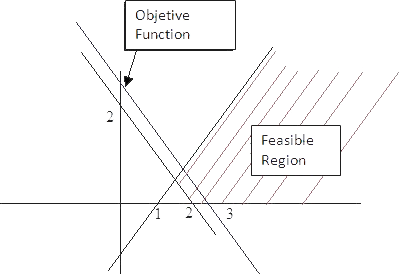# Linear Programming Samples

### Unbounded solution Sample

If we consider
Maximize (x + y)
Subject to
x - y ≥ 1
x + y ≥ 2
x, y ≥ 0

The feasible region is as followsIn this case, you can see we can move as much as we want the objective function in the growing sense of x and y coordinates without leaving the feasible region
Therefore, objective function can grow too into feasible region, so we are in an unbounded solution case for this problem.

# Was useful? want add anything?

Post here

### Abdi:

2013-02-24 22:26:02
very useful. Thank you so much

Post here